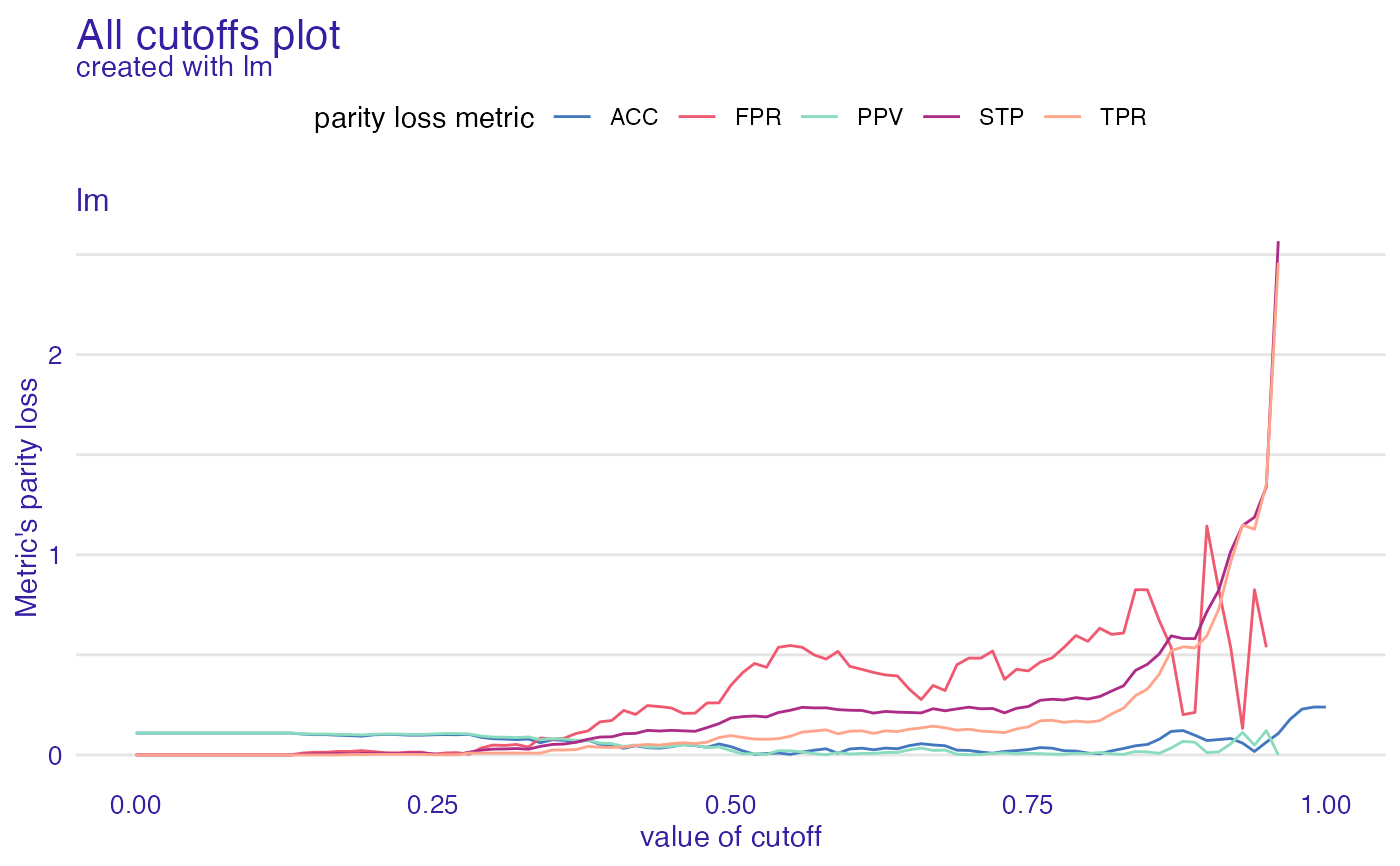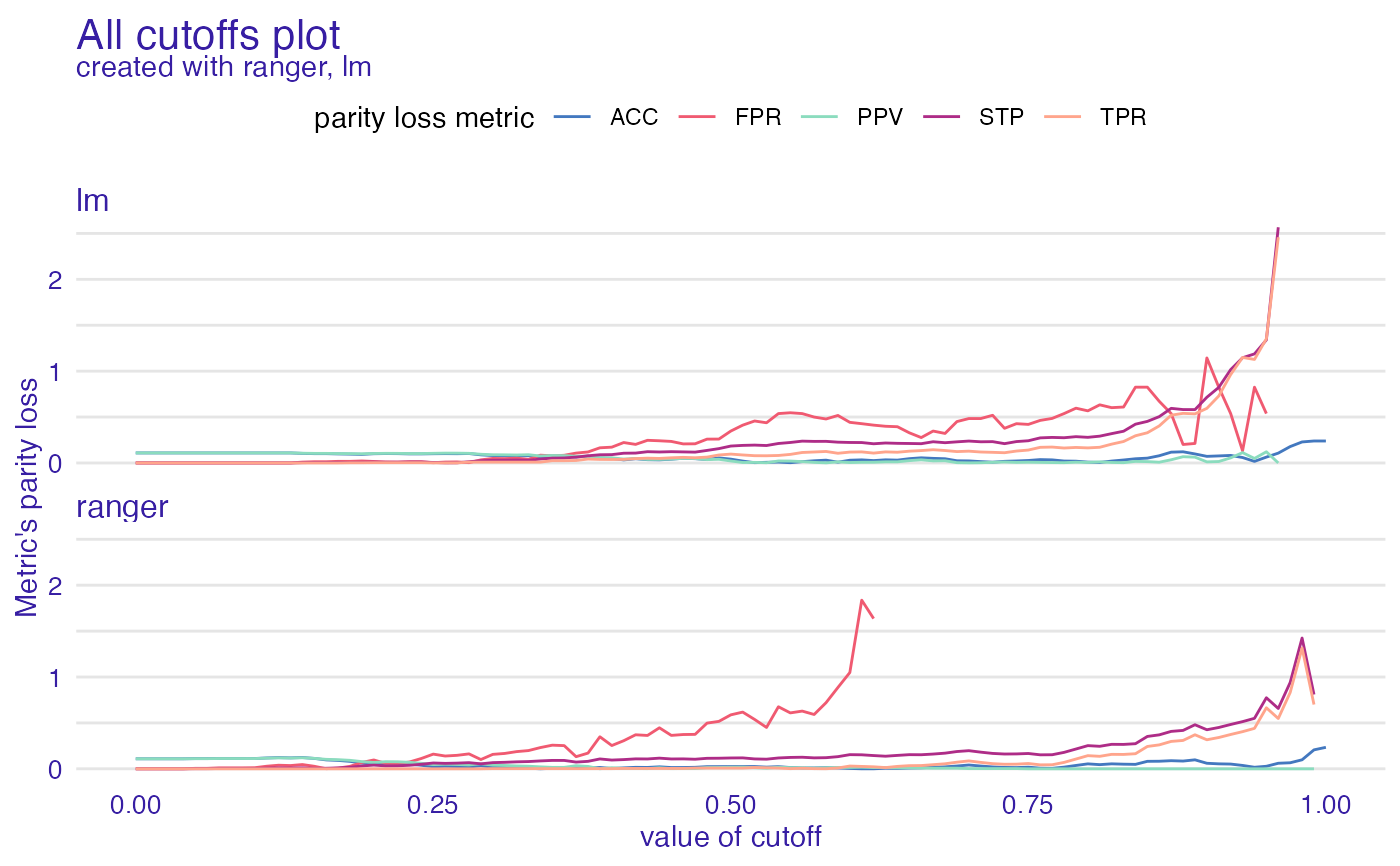Create all_cutoffs object and see how with the change of cutoffs parity loss of fairness metrics changes. Value of cutoff changes equally for all subgroups. User can pick which fairness metrics to create the object with via fairness_metrics vector.

all_cutoffs(
x,
grid_points = 101,
fairness_metrics = c("ACC", "TPR", "PPV", "FPR", "STP")
)

Arguments

x object of class fairness_object numeric, grid for cutoffs to test. Number of points between 0 and 1 spread evenly character, name of parity_loss metric or vector of multiple metrics names. Full names can be found in fairness_check documentation.

Value

all_cutoffs object, data.frame containing information about label, metric and parity_loss at particular cutoff

Examples

data("german")

y_numeric <- as.numeric(german$Risk) - 1 lm_model <- glm(Risk ~ ., data = german, family = binomial(link = "logit") ) explainer_lm <- DALEX::explain(lm_model, data = german[, -1], y = y_numeric) #> Preparation of a new explainer is initiated #> -> model label : lm ( default ) #> -> data : 1000 rows 9 cols #> -> target variable : 1000 values #> -> predict function : yhat.glm will be used ( default ) #> -> predicted values : No value for predict function target column. ( default ) #> -> model_info : package stats , ver. 4.1.1 , task classification ( default ) #> -> predicted values : numerical, min = 0.1369187 , mean = 0.7 , max = 0.9832426 #> -> residual function : difference between y and yhat ( default ) #> -> residuals : numerical, min = -0.9572803 , mean = 1.940006e-17 , max = 0.8283475 #> A new explainer has been created! fobject <- fairness_check(explainer_lm, protected = german$Sex,
privileged = "male"
)
#> Creating fairness classification object
#> -> Privileged subgroup		: character ( Ok  )
#> -> Protected variable		: factor ( Ok  )
#> -> Cutoff values for explainers	: 0.5 ( for all subgroups )
#> -> Fairness objects		: 0 objects
#> -> Checking explainers		: 1 in total (  compatible  )
#> -> Metric calculation		: 13/13 metrics calculated for all models
#>  Fairness object created succesfully

ac <- all_cutoffs(fobject)
plot(ac)
#> Warning: Removed 17 row(s) containing missing values (geom_path).# \donttest{
rf_model <- ranger::ranger(Risk ~ .,
data = german,
probability = TRUE,
num.trees = 100,
seed = 1
)

explainer_rf <- DALEX::explain(rf_model,
data = german[, -1],
y = y_numeric
)
#> Preparation of a new explainer is initiated
#>   -> model label       :  ranger  (  default  )
#>   -> data              :  1000  rows  9  cols
#>   -> target variable   :  1000  values
#>   -> predict function  :  yhat.ranger  will be used (  default  )
#>   -> predicted values  :  No value for predict function target column. (  default  )
#>   -> model_info        :  package ranger , ver. 0.13.1 , task classification (  default  )
#>   -> predicted values  :  numerical, min =  0.04380952 , mean =  0.6969957 , max =  1
#>   -> residual function :  difference between y and yhat (  default  )
#>   -> residuals         :  numerical, min =  -0.7478095 , mean =  0.003004293 , max =  0.708996
#>   A new explainer has been created!

fobject <- fairness_check(explainer_rf, fobject)
#> Creating fairness classification object
#> -> Privileged subgroup		: character ( from first fairness object  )
#> -> Protected variable		: factor ( from first fairness object  )
#> -> Cutoff values for explainers	: 0.5 ( for all subgroups )
#> -> Fairness objects		: 1 object (  compatible  )
#> -> Checking explainers		: 2 in total (  compatible  )
#> -> Metric calculation		: 10/13 metrics calculated for all models ( 3 NA created )
#>  Fairness object created succesfully

ac <- all_cutoffs(fobject)

plot(ac)
#> Warning: Removed 41 row(s) containing missing values (geom_path).# }